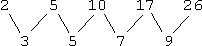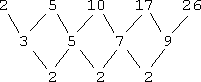Search

Finding the Next Number in a Sequence:
The Method of Common Differences
(page 1 of 7)

Sections: Common differences, Recursions, General examples, Non-math "sequences"When faced with a sequence for which you need to find missing values or the next few values, you need first to look at it and see if you can get a "feel" for what is going on. For instance:

• Find the next number in the following sequence:  1, 4, 9, 16, 25,....

I notice that 12 = 1, 22 = 4, 32 = 9, 42 = 16, and 52 = 25. So it looks as though the pattern here is squaring. That is, for the first term (the 1-st term), it looks like they squared 1; for the second term (the 2-nd term), they squared 2; for the third term (the 3-rd term), they squared 3; and so on. For the n-th term ("the enn-eth term"), they will probably want me to square n. In particular, for the sixth term, they will probably want me to square 6.

The next number in the sequence is 62 = 36.

Why is the answer only "probably" the square of six? Because "the right answer" is nothing more than whatever answer the author had in mind when he designed the exercise; you might "see" a completely different pattern that he hadn't intended. But as long as your answer is something that you can mathematically (or at least logically) justify, your answer should be acceptable, even if it isn't "right".

• Find the next number in the following sequence:  1, 4, 27, 256,....

This pattern looks similar to the previous sequence, but with 11 = 1, 22 = 4, 33 = 27, and 44 = 256. The pattern seems to be that the n-th term is of the form nn.

Then the next term, being the fifth term (n = 5) is 55 = 3125.

• Find the next number in the following sequence:  2, 5, 10, 17, 26,....

I have to be more clever to figure out the pattern on this sequence. Each term is 1 more than a square. That is, the pattern is given by:

 n n2 n2 + 1 1 1 2 2 4 5 3 9 10 4 16 17 5 25 26

So the n-th term is given by n2 + 1. Then the sixth term is:

62 + 1 = 36 + 1 = 37

Note that these two sequences were generated by the polynomials n2 and n2 + 1. But what if the sequence is generated by a more complicated polynomial? How would you figure it out then? There is a method, and I'll demonstrate it by re-doing the second sequence above, where we already know what the pattern is.   Copyright © Elizabeth Stapel 2002-2011 All Rights Reserved

• Find the next number in the following sequence:  2, 5, 10, 17, 26,...., and provide a formula for the n-th term.

To find the pattern, I will list the numbers, and find the differences for each pair of numbers. That is, I will subtract the numbers in pairs (the first from the second, the second from the third, and so on), like this:Since these values, the "first differences", are not all the same value, I'll continue subtracting:Since these values, the "second differences", are all the same value, then I can stop. It isn't important what the second difference is (in this case, "2"); what is important is that the second differences are the same, because this tells me that the polynomial for this sequence of values is a quadratic. (Once you've studied calculus, you'll be able to understand why this is so. For now, just trust me that this works.) Since the formula for the terms is a quadratic, then I know that it is of the form:

an2 + bn + c

...for some numbers a, b, and c. Now I have to find those numbers. How? By plugging in some of the values from the sequence, and then solving the resulting system of equations. For instance, I know that the first term (that is, the term when n = 1) is 2, so I'll plug in 1 for n and 2 for the value:

a(1)2 + b(1) + c = a + b + c = 2

The second term (that is, the term when n = 2) is 5, so:

a(2)2 + b(2) + c = 4a + 2b + c = 5

The third term (that is, the term when n = 3) is 10, so:

a(3)2 + b(3) + c = 9a + 3b + c = 10

This gives me a system of three equations in three unknowns, which I can solve. You can use whatever method you like, including using matrices in your graphing calculator:(Remember that calculators suffer from round-off error. When you get a result like "1.3E13", which represents "0.00000000000013", you should be aware that, in this context, this means "zero".)

Then a = 1, b = 0, and c = 1, so the formula is:

1n2 + 0n + 1 = n2 + 1

...just as I had determined before, and the sixth term is:

next term: 62 + 1 = 36 + 1 = 37
formula for the n-th term: n2 + 1

You can simplify your computations somewhat by using a formula for the leading coefficient of the sequence's polynomial. The coefficient of the first term of the polynomial will be equal to the common difference divided by the factorial of the polynomial's degree. In the example above, the common difference was 2 and the degree was 2, so the leading coefficient was 2 ÷ 2! = 2 ÷ 2 = 1. I'm not aware of any formulas for the other coefficients.

Top  |  1 | 2 | 3 | 4 | 5 | 6 | 7  |  Return to Index  Next >>

 Cite this article as: Stapel, Elizabeth. "Finding the Next Number in a Sequence: The Method of Common Differences."     Purplemath. Available from https://www.purplemath.com/modules/nextnumb.htm.     Accessed [Date] [Month] 2016

Study Skills Survey

Tutoring from Purplemath
Find a local math tutor